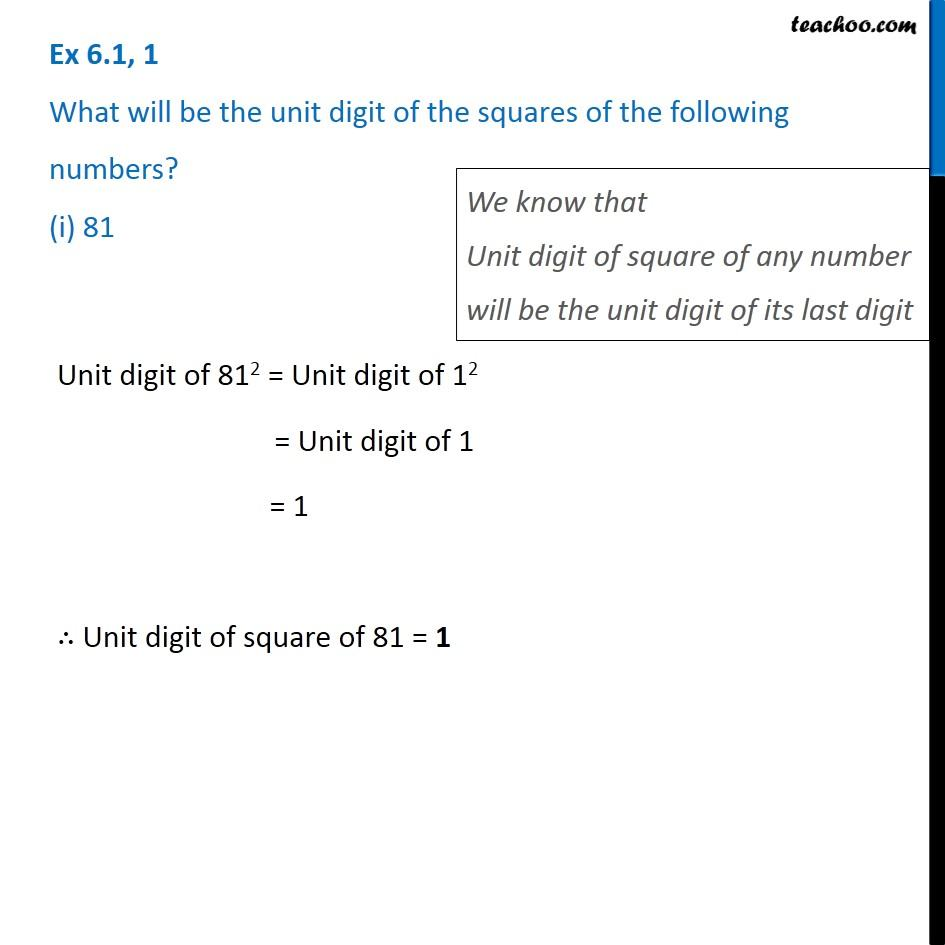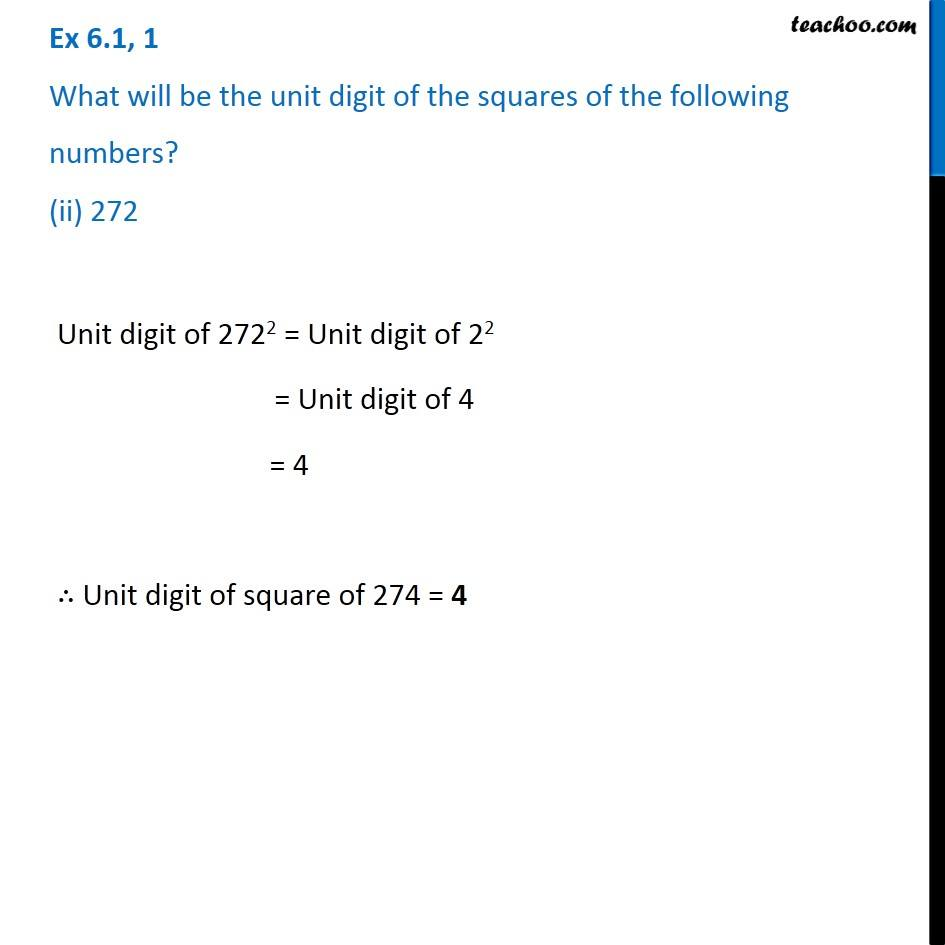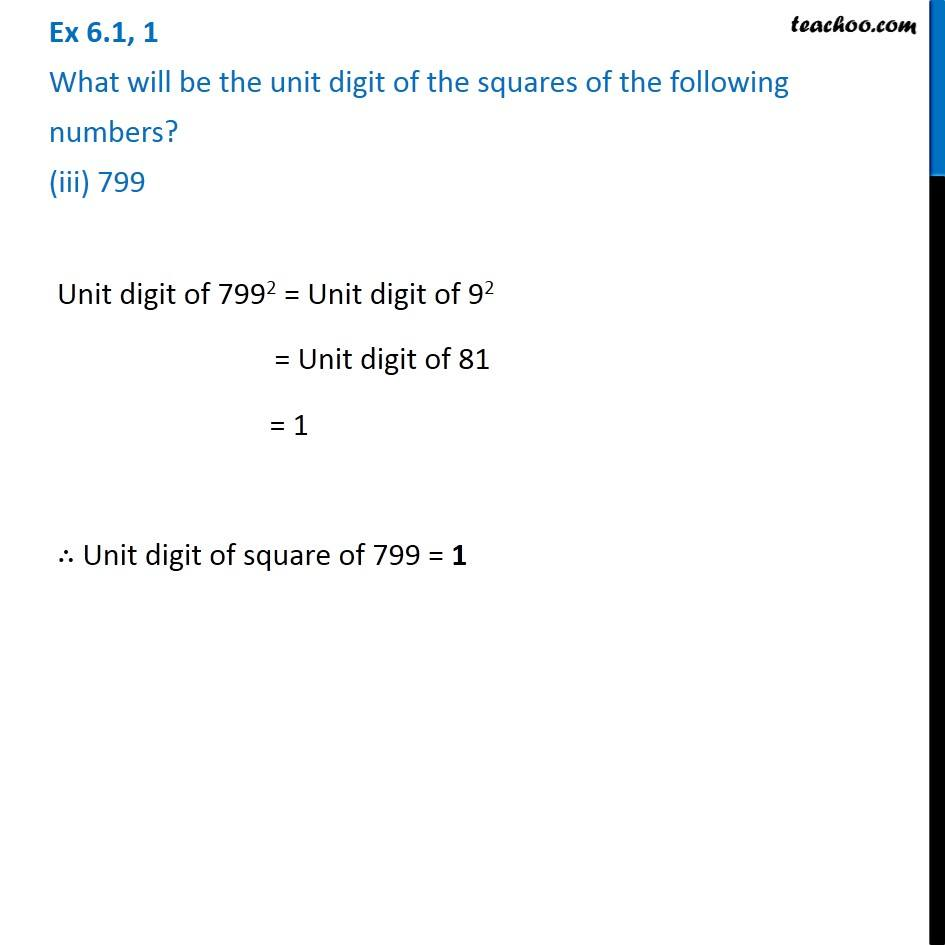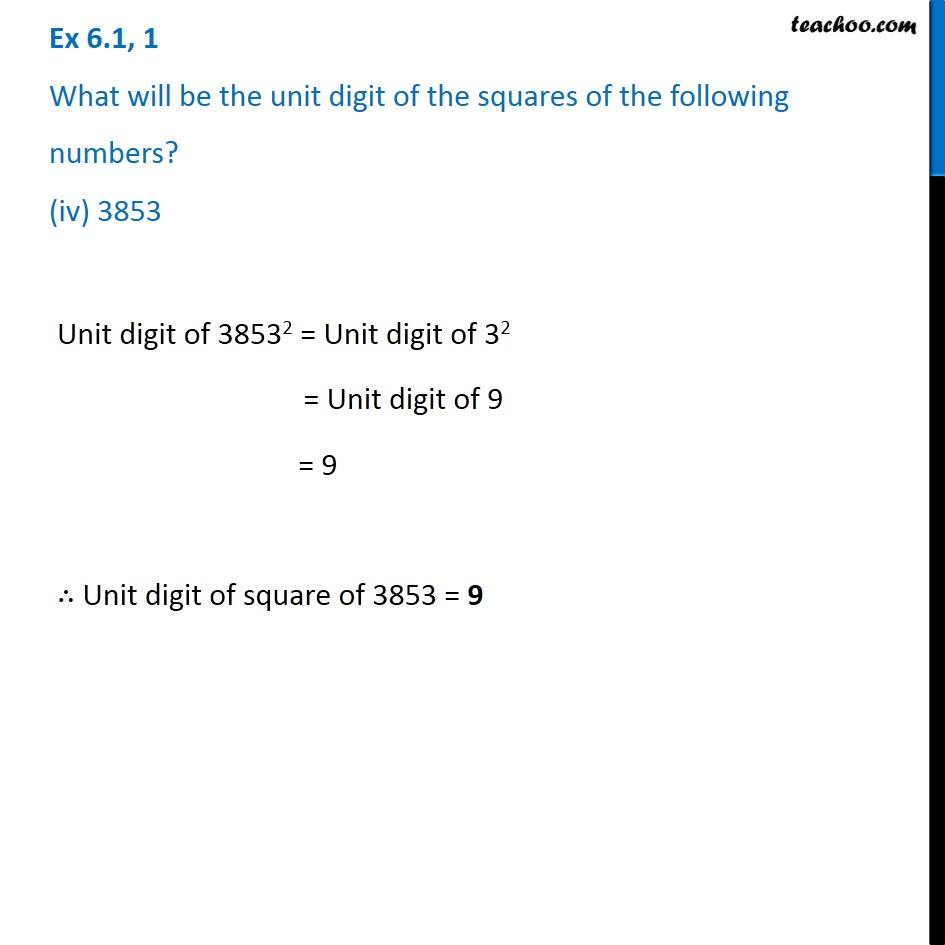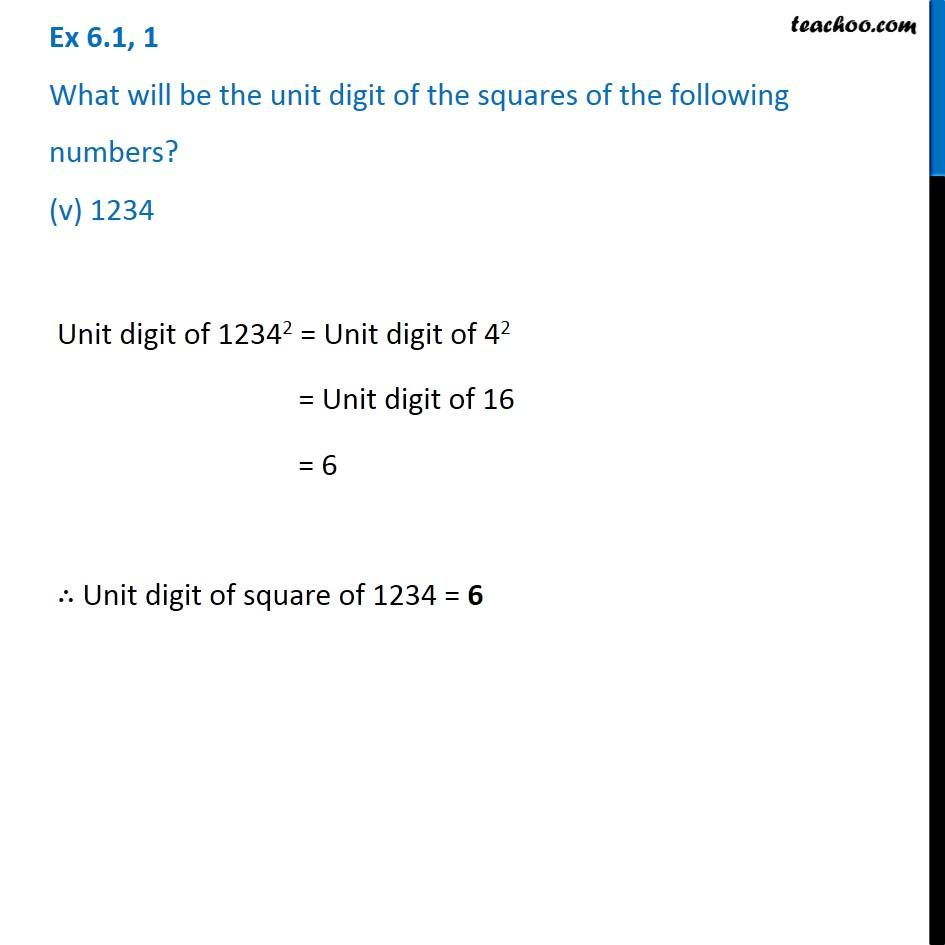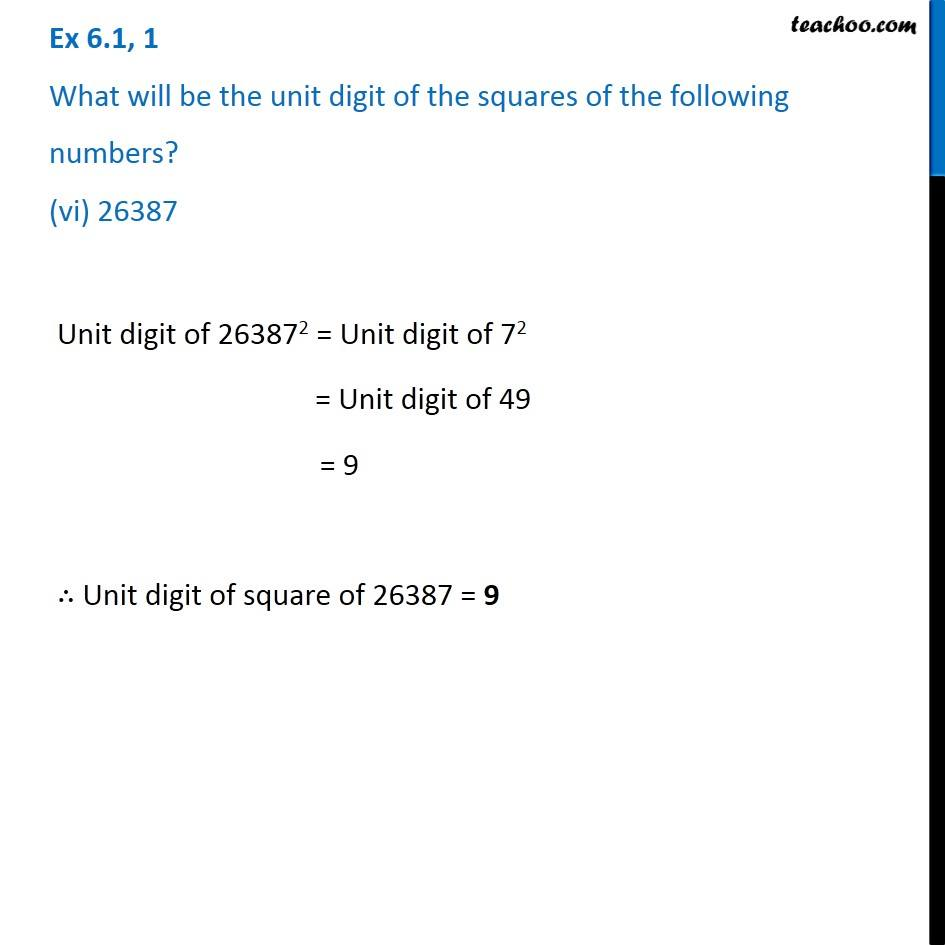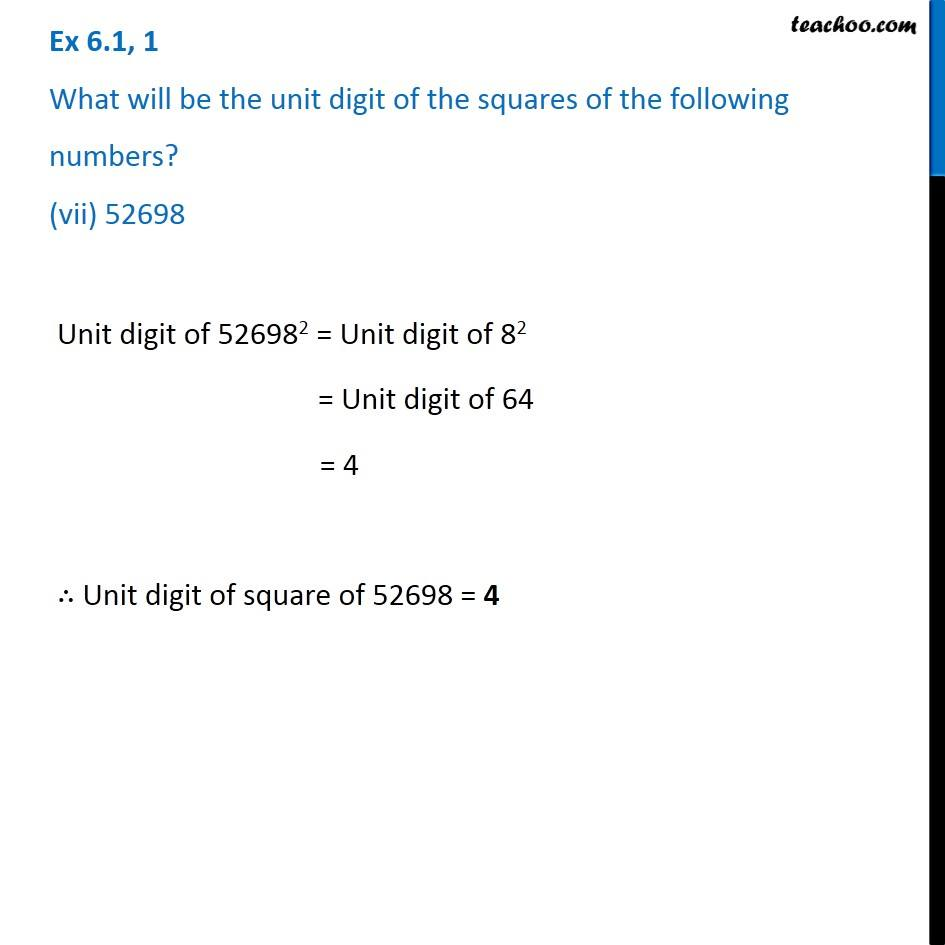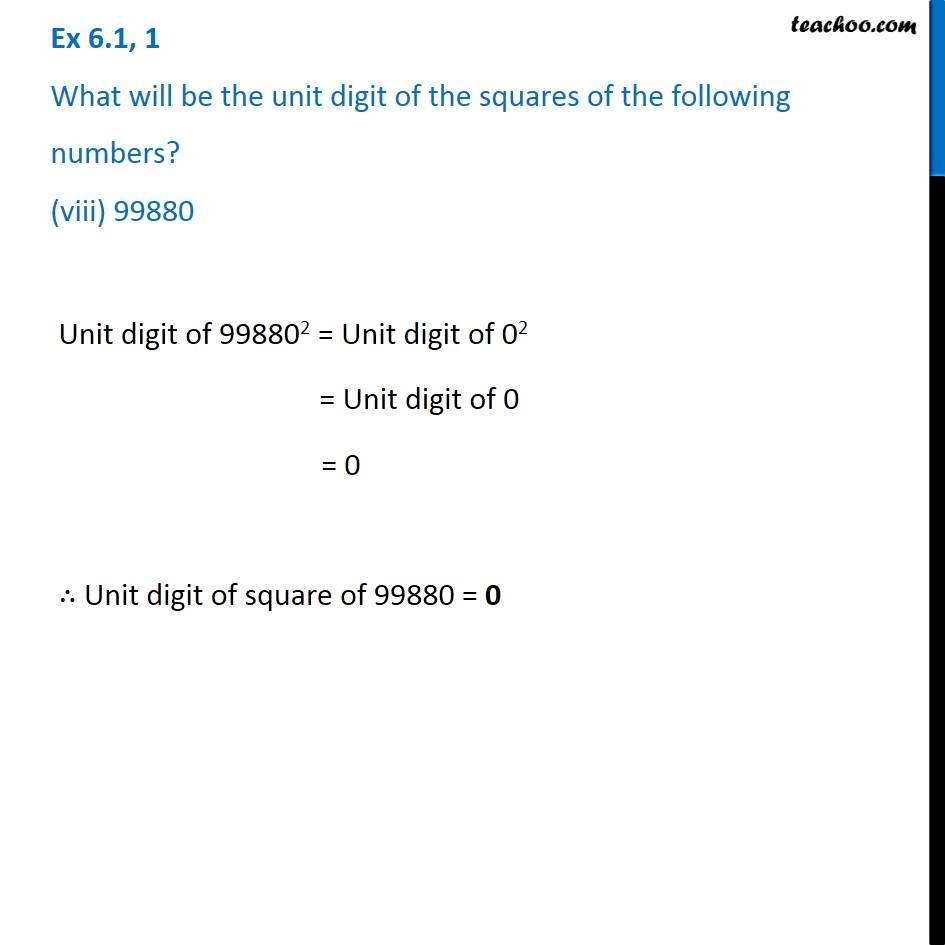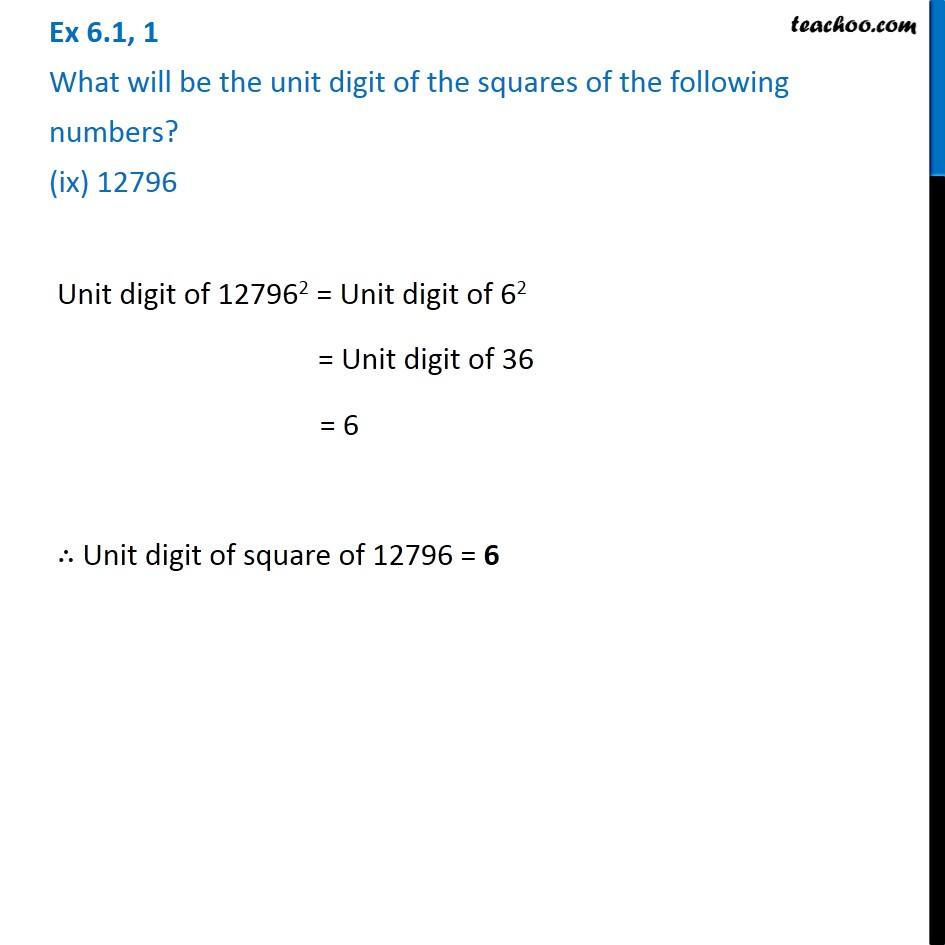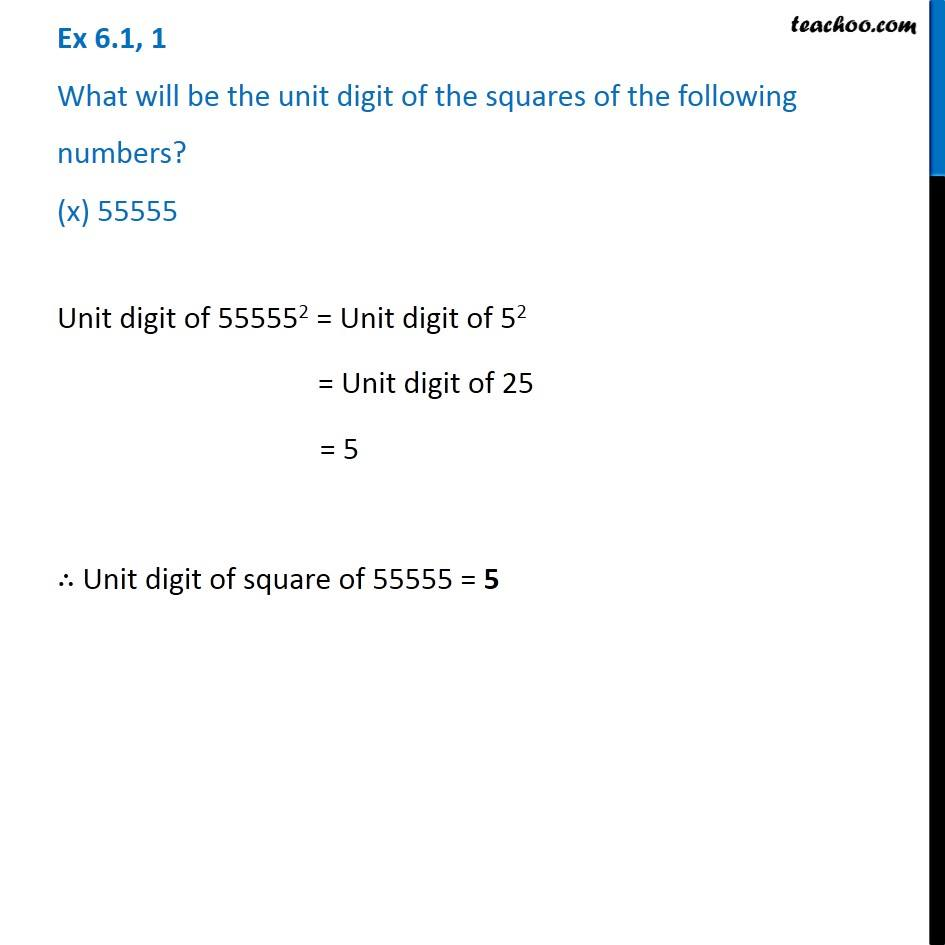Subscribe to our Youtube Channel - https://you.tube/teachoo

1. Chapter 6 Class 8 Squares and Square Roots
2. Serial order wise
3. Ex 6.1

Transcript

Ex 6.1, 1 What will be the unit digit of the squares of the following numbers? (i) 81We know that Unit digit of square of any number will be the unit digit of its last digit Unit digit of 812 = Unit digit of 12 = Unit digit of 1 = 1 ∴ Unit digit of square of 81 = 1 Ex 6.1, 1 What will be the unit digit of the squares of the following numbers? (ii) 272Unit digit of 2722 = Unit digit of 22 = Unit digit of 4 = 4 ∴ Unit digit of square of 274 = 4 Ex 6.1, 1 What will be the unit digit of the squares of the following numbers? (iii) 799Unit digit of 7992 = Unit digit of 92 = Unit digit of 81 = 1 ∴ Unit digit of square of 799 = 1 Ex 6.1, 1 What will be the unit digit of the squares of the following numbers? (iv) 3853Unit digit of 38532 = Unit digit of 32 = Unit digit of 9 = 9 ∴ Unit digit of square of 3853 = 9 Ex 6.1, 1 What will be the unit digit of the squares of the following numbers? (v) 1234Unit digit of 12342 = Unit digit of 42 = Unit digit of 16 = 6 ∴ Unit digit of square of 1234 = 6 Ex 6.1, 1 What will be the unit digit of the squares of the following numbers? (vi) 26387Unit digit of 263872 = Unit digit of 72 = Unit digit of 49 = 9 ∴ Unit digit of square of 26387 = 9 Ex 6.1, 1 What will be the unit digit of the squares of the following numbers? (vii) 52698Unit digit of 526982 = Unit digit of 82 = Unit digit of 64 = 4 ∴ Unit digit of square of 52698 = 4 Ex 6.1, 1 What will be the unit digit of the squares of the following numbers? (viii) 99880Unit digit of 998802 = Unit digit of 02 = Unit digit of 0 = 0 ∴ Unit digit of square of 99880 = 0 Ex 6.1, 1 What will be the unit digit of the squares of the following numbers? (ix) 12796Unit digit of 127962 = Unit digit of 62 = Unit digit of 36 = 6 ∴ Unit digit of square of 12796 = 6 Ex 6.1, 1 What will be the unit digit of the squares of the following numbers? (x) 55555Unit digit of 555552 = Unit digit of 52 = Unit digit of 25 = 5 ∴ Unit digit of square of 55555 = 5

Ex 6.1